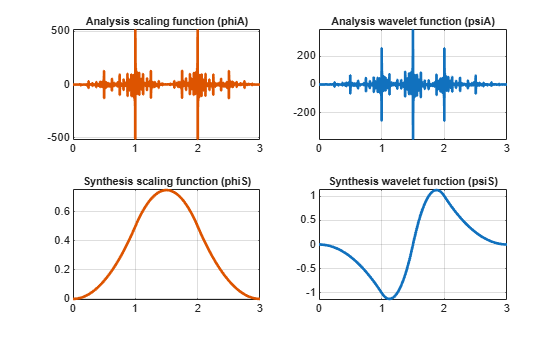Main Content

# bswfun

Biorthogonal scaling and wavelet functions

## Syntax

```[PHIS,PSIS,PHIA,PSIA,XVAL] = bswfun(LoD,HiD,LoR,HiR) bswfun(LoD,HiD,LoR,HiR,ITER) bswfun(LoD,HiD,LoR,HiR,'plot') bswfun(LoD,HiD,LoR,HiR,ITER,'plot') bswfun(LoD,HiD,LoR,HiR,'plot',ITER) ```

## Description

`[PHIS,PSIS,PHIA,PSIA,XVAL] = bswfun(LoD,HiD,LoR,HiR)` returns approximations on the grid `XVAL` of the two pairs of biorthogonal scaling and wavelet functions. `PHIS` and `PSIS` are the scaling and wavelet functions constructed from the decomposition filters, `LoD` and `HiD`. `PHIA` and `PSIA` are the scaling and wavelet functions constructed from the reconstruction filters, `LoR` and `HiR`.

`bswfun(LoD,HiD,LoR,HiR,ITER)` computes the two pairs of scaling and wavelet functions using ITER iterations.

`bswfun(LoD,HiD,LoR,HiR,'plot')` or `bswfun(LoD,HiD,LoR,HiR,ITER,'plot')` or `bswfun(LoD,HiD,LoR,HiR,'plot',ITER)` computes and plots the functions.

## Examples

collapse all

This example shows how to obtain the biorthogonal scaling and wavelet functions corresponding to a lifting scheme. Obtain the lifting scheme for the CDF 3/1 wavelet.

`lscdf = liftwave('cdf3.1');`

Display the lifting scheme, which consists of two primal and one dual step.

```Sc = displs(lscdf); Sc```
```Sc = 6x50 char array 'lscdf = {... ' ''p' [ -0.33333333] [-1] ' ''d' [ -0.37500000 -1.12500000]  ' ''p' [ 0.44444444]  ' '[ 2.12132034] [ 0.47140452] [] ' '}; ' ```

Obtain the decomposition and reconstruction filters from the lifting scheme.

`[LoD,HiD,LoR,HiR] = ls2filt(lscdf);`

Visualize the scaling and wavelet function and their duals.

`bswfun(LoD,HiD,LoR,HiR,'plot');`## Algorithms

This function uses the cascade algorithm.

## See Also

Introduced before R2006a

## SupportGet trial now